### IMO Shortlist 2008 problem A4

Kvaliteta:
Avg: 1,0
Težina:
Avg: 7,0
For an integer$m$, denote by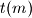$t(m)$ the unique number in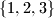$\{1, 2, 3\}$ such that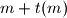$m + t(m)$ is a multiple of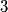$3$. A function$f: \mathbb{Z}\to\mathbb{Z}$ satisfies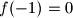$f( - 1) = 0$,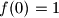$f(0) = 1$,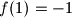$f(1) = - 1$ and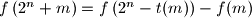$f\left(2^{n} + m\right) = f\left(2^n - t(m)\right) - f(m)$ for all integers$m$,$n\ge 0$ with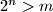$2^n > m$. Prove that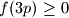$f(3p)\ge 0$ holds for all integers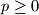$p\ge 0$.

Proposed by Gerhard Woeginger, Austria
Izvor: Međunarodna matematička olimpijada, shortlist 2008